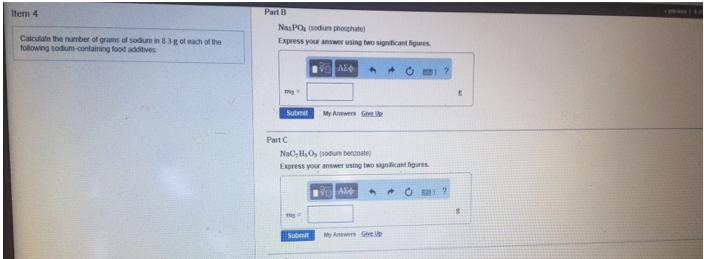# Problem: Calculate the number of grams of sodium in 8.3 g of each of the following sodium-containing food additives.Part BNa2PO4 (sodium phosphate) Express your answer using two significant figures.Part CNaC7H5O2 (sodium benzonate)Express your answer using two significant figures.

###### FREE Expert Solution
97% (497 ratings)###### Problem Details

Calculate the number of grams of sodium in 8.3 g of each of the following sodium-containing food additives.

Part B

Na2PO4 (sodium phosphate)

Part C

NaC7H5O2 (sodium benzonate)